# 9.2.3 Loci in Two Dimensions, PT3 Focus Practice

Question 7:
Diagram below in the answer space shows a circle with centre O drawn on a grid of equal squares with sides of 1 unit. POQ is a diameter of the circle.
W, X and Y are three moving points inside the circle.
(a) W is the point which moves such that it is constantly 4 units from the point O. Describe fully the locus of W.
(b) On the diagram, draw,
(i) the locus of the point X which moves such that its distance is constantly 3 units from the line PQ,
(ii) the locus of the point Y which moves such that it is equidistant from the point P and the point Q.
(c) Hence, mark with the symbol $\otimes$ the intersection of the locus of X and the locus of Y.

(b)(i),(ii) and (c)Solution:
(a) The locus of W is a circle with the centre O and a radius of 4 units.

(b)(i),(ii) and (c)Question 8:
The diagram in the answer space shows two squares ABCD and CDEF each of sides 4 cm. K is a point on the line CD. W, X and Y are three moving points in the diagram.
(a) Point W moves such that it is always equidistant from the straight lines AB and EF. By using the letters in diagram, state the locus of W.
(b) On the diagram, draw
(i) the locus X such that it is always 2 cm from the straight line ACE,
(ii) the locus of Y such that KY = KC.
(c) Hence, mark with the symbol $\otimes$ the intersection of the locus of X and the locus of Y.

(b)(i), (ii) and (c)Solution:
(a) The locus of W is the line CD.

(b)(i),(ii) and (c)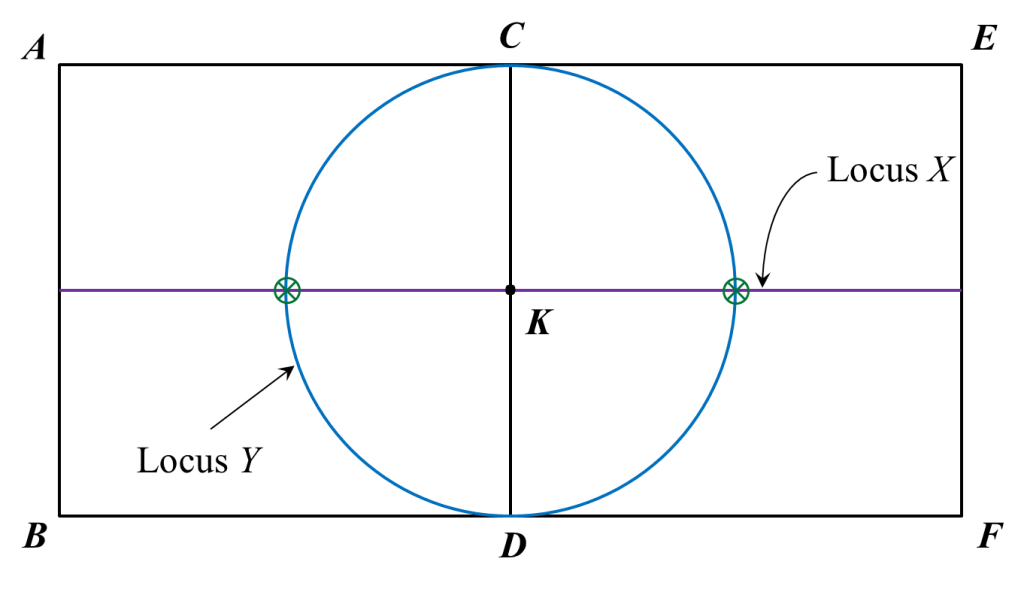Question 9:
Diagram below shows a Cartesian plane.
Draw the locus of X, Y and Z.
(a) X is a point which moves such that its distance is constantly 4 units from the origin.
(b) Y is a point which moves such that it is always equidistant from point K and point L.
(c) Z is a point which moves such that it is always equidistant from lines KM and y-axis.Solution: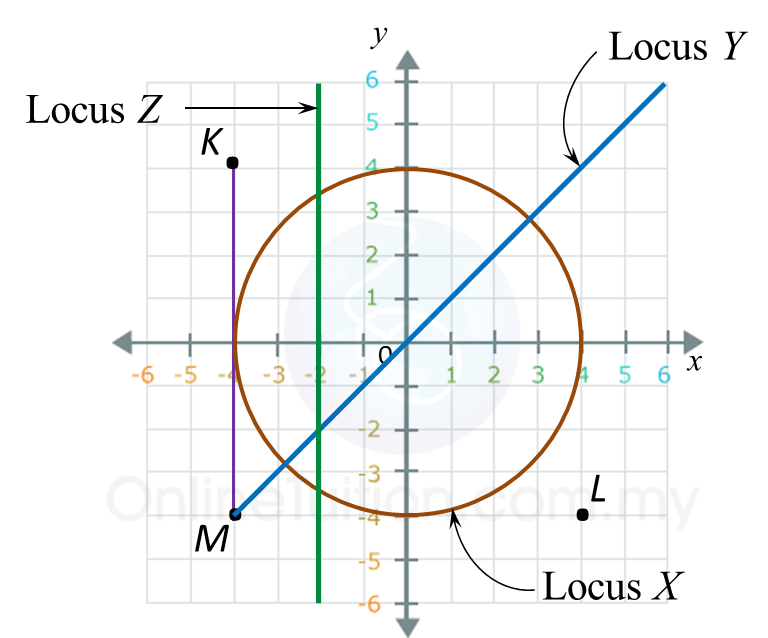# 9.2.2 Loci in Two Dimensions, PT3 Focus Practice

9.2.2 Loci in Two Dimensions, PT3 Focus Practice

Question 4:
Diagram below in the answer space shows a quadrilateral ABCD drawn on a grid of equal squares with sides of 1 unit.
X, Y and Z are three moving points inside the quadrilateral ABCD.
(a) X is the point which moves such that it is always equidistant from point B and point D.
By using the letters in diagram, state the locus of X.
(b) On the diagram, draw,
(i) the locus of the point Y such that it is always 6 units from point A,
(ii) the locus of the point Z which moves such that its distance is constantly 3 units from the
line AB.
(c) Hence, mark with the symbol ⊗ the intersection of the locus of Y and the locus of Z.

:
(b)(i),(ii) and (c)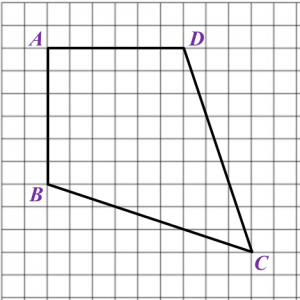Solution
:
(a) The locus of X is the line AC.

(b)(i),(ii) and (c)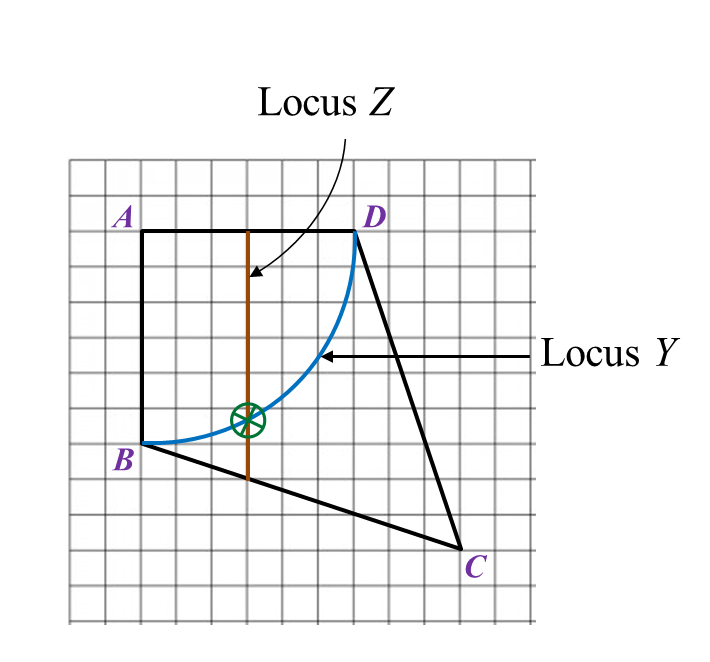Question 5:
Diagram in the answer space below shows a square ABCD. E, F, G and H are the midpoints of straight lines AD, AB, BC and CD respectively. W, X and Y are moving points in the square.
On the diagram,
(a) draw the locus of the point W which moves such that it is always equidistant from point AD and BC.
(b)   draw the locus of the point X which moves such that XM = MG.
(c) draw the locus of point Y which moves such that its distance is constantly 6 cm from point C.
(d)   Hence, mark with the symbol ⊗  the intersection of the locus of W and the locus of Y.

:
(a), (b), (c) and (d)Solution
: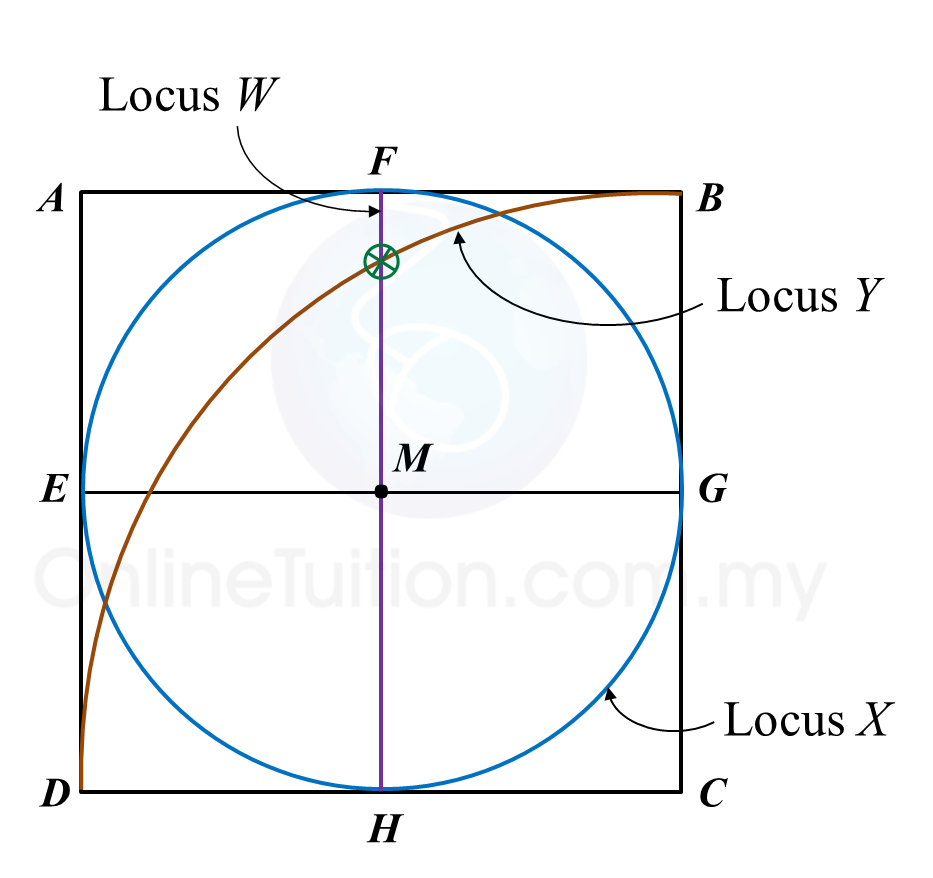Question 6:
Diagram in the answer space below shows a polygon ABCDEF drawn on a grid of squares with sides of 1 unit. X, Y and Z are the points that move in the polygon.
(a) X is a point which moves such that it is equidistant from point B and point F.
By using the letters in diagram, state the locus of X.
(b) On the diagram, draw
(i) the locus of the point Y which moves such that it is always parallel and equidistant from the straight lines BA and CD.
(ii) the locus of point Z which moves such that its distance is constantly 6 units from the point A.
(c) Hence, mark with the symbol $\otimes$ the intersection of the locus of Y and the locus of Z.

(b)(i), (ii) and (c)Solution:
(a) The locus of X is AD.

(b)(i),(ii) and (c)# 9.2.1 Loci in Two Dimensions, PT3 Focus Practice

9.2.1 Loci in Two Dimensions, PT3 Focus Practice

Question 1:
Diagram below in the answer space shows a square PQRS with sides of 6 units drawn on a grid of equal squares with sides of 1 unit. W, X and Y are three moving points inside the square.
(a) W is the point which moves such that it is always equidistant from point P and point R.
By using the letters in diagram, state the locus of W.
(b) On the diagram, draw,
(i) the locus of the point X which moves such that it is always equidistant from the straight lines PQ and PS,
(ii) the locus of the point Y which moves such that its distance is constantly 2 units from point K.
(c) Hence, mark with the symbol ⊗ the intersection of the locus of X and the locus of Y.

:
(b)(i),(ii)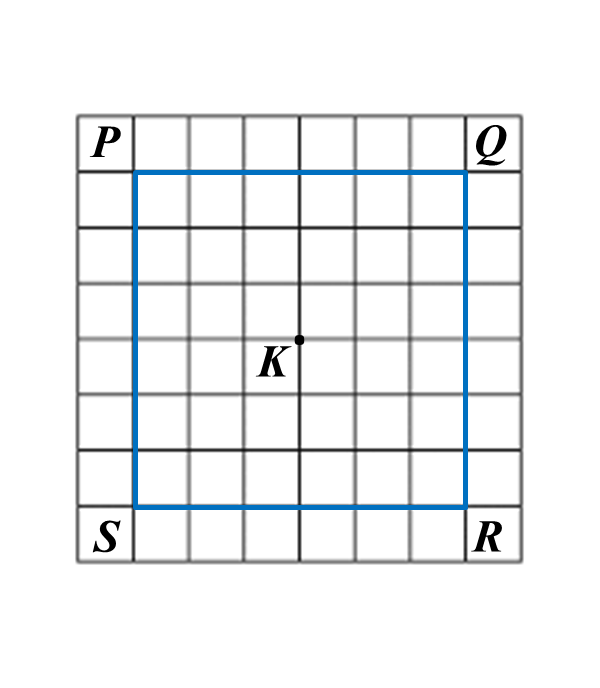Solution:
(a) QS

(b)(i),(ii)
(c)Question 2:
Diagram in the answer space below, shows a regular pentagon PQRST. W, X and Y are moving points which move in the pentagon.
On the diagram,
(a) draw the locus of the point W which moves such that it is always equidistant from point R and S.
(b) draw the locus of the point X which moves such that XR = RS.
(c) draw the locus of point Y which moves such that its distance is constantly 3 cm from the line SR.
(d) hence, mark with the symbol ⊗ the intersection of the locus of W and the locus of X.

:
(a), (b), (c) and (d)Solution:
(a), (b), (c) and (d)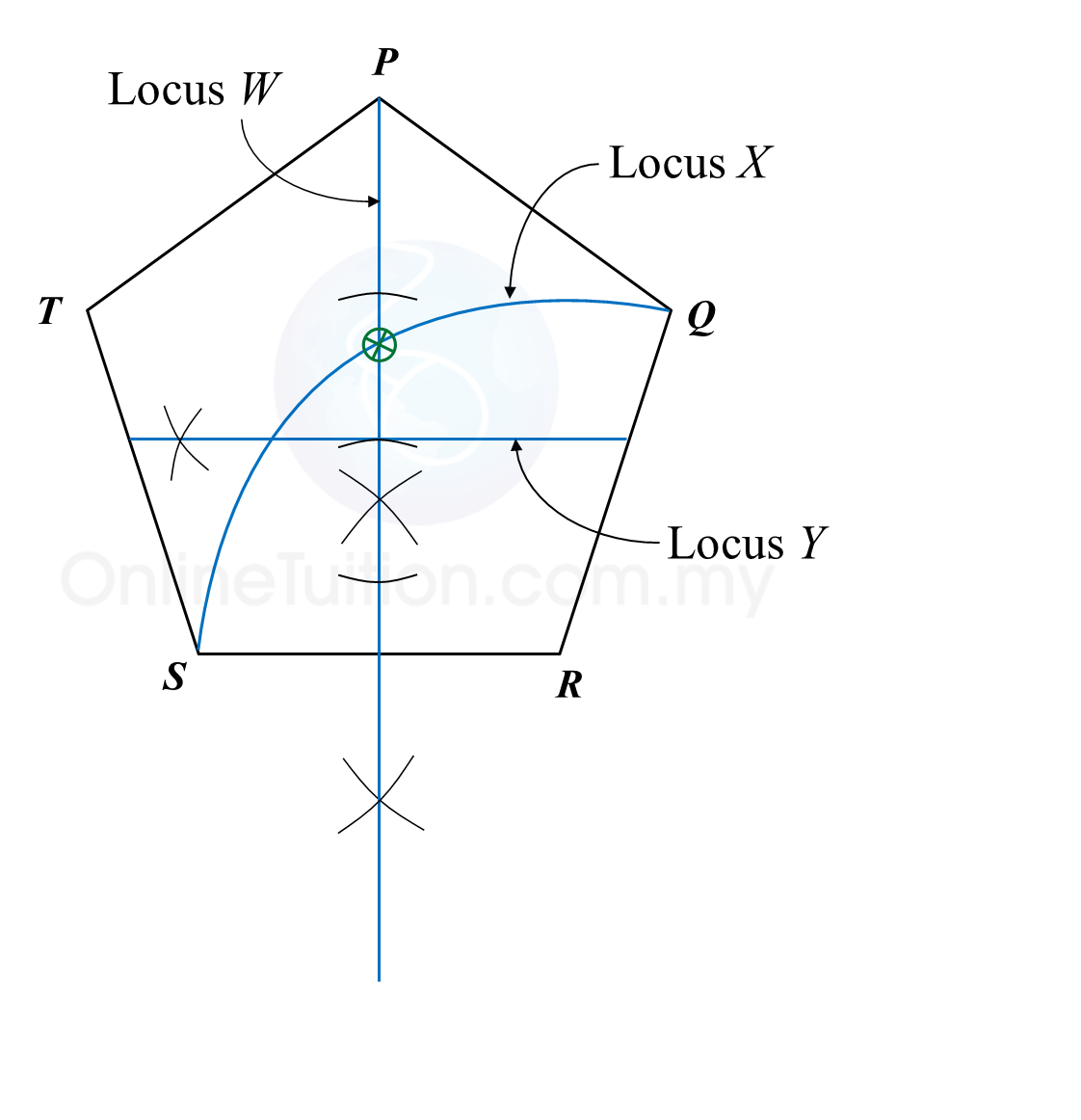Question 3:
Diagram in the answer space below shows a polygon. X and Y are two moving points in the polygon.
(a) On the diagram, draw
(i) the locus of the point X such that XQ = XR.
(ii) the locus of the point Y such that YQ = QR.
(b) Hence, mark with the symbol $\otimes$ the intersection of the locus of X and the locus of Y.

(a)(i), (ii) and (b)Solution:
(a)(i), (ii) and (b)# 8.2.3 Coordinates, PT3 Focus Practice

Question 11:
Diagram below shows the route of a Negaraku Run.The route of male participants is PQUT while the route for female participants is QRST. QR is parallel to UT whereas UQ is parallel to SR.
(a) Given the distance between point Q and point R is 9 km, state the coordinates of point R.
(b) It is given that the male participants start the run at point P and the female participants at point Q.
Find the difference of distance, in km, between the male and female participants.

Solution:
(a)
R = (9, 1)

(b)
Route of male participants
= PQ + QU + UT
= 10 + 7 + 7
= 24 km

Route of female participants
= QR + RS + ST
= 9 + 7 + 2
= 18 km

Difference in distance
= 24 – 18
= 6 km

Question 12:
Diagram below shows a parking lot in the shape of trapezium, RSTU. Two coordinates from the trapezium vertices are R(–30, –4) and S(20, –4).(a) Given the distance between vertex S and vertex T is 22 units.
State the coordinates of vertex T.
(b) Given the area of the parking lot is 946 unit2, find the coordinates of U.

Solution:
(a)
y-coordinates of T = –4 + 22 = 18
T = (20, 18)

(b)

# 8.2.1 Coordinates, PT3 Focus Practice

8.2.1 Coordinates, PT3 Focus Practice

Question 1
:
In diagram below, Q is the midpoint of the straight line PR.
The value of is

Solution
:
$\begin{array}{l}\frac{2+m}{2}=5\\ 2+m=10\\ \text{}m=8\end{array}$

Question 2:
In diagram below, P and Q are points on a Cartesian plane.

If M is the midpoint of PQ, then the coordinates of M are

Solution:

Question 3:
Find the distance between (–4, 6) and (20, –1).

Solution
:

Question 4:
Diagram shows a straight line PQ on a Cartesian plane.

Calculate the length, in unit, of PQ.

Solution
:
PS = 15 – 3 = 12 units
SQ = 8 – 3 = 5 units
By Pythagoras’ theorem,
PQ= PS2 + SQ2
= 122+ 52
PQ = √169
= 13 units

Question 5:
The diagram shows an isosceles triangle STU.

Given that ST = 5 units, the coordinates of point are

Solution
:

For an isosceles triangle STU, M is the midpoint of straight line TU.
Point M = (1, 0)

MT = 4 – 1 = 3 units
By Pythagoras’ theorem,
SM= ST2MT2
= 52 – 32
= 25 – 9
= 16
SM = √16
= 4
Therefore, point S = (1, 4).

# 8.1 Coordinates

8.1 Coordinates

8.1.1 Coordinates
1. The Cartesian coordinate system is a number plane with a horizontal line (x-axis) drawn at right angles to a vertical line (y-axis), intersecting at a point called origin.
2. It is used to locate the position of a point in reference to the x-axis and y-axis.

3. The coordinate of any point are written as an ordered pair (x, y).  The first number is the x-coordinate and the second number is the y-coordinate of the point.

Example:

The coordinates of points A and B are (3, 4) and (–5, –2) respectively.
This means that point A is located 3 units from they-axis and 4 units from the x-axis, whereas point B is located 5 units on the left from the y-axis and 2 units from the x-axis.

4. The coordinate of the origin O is (0, 0).

8.1.2 Scales for the Coordinate Axes
1. The scale for an axis is the number of units represented by a specific length along the axes.

2.
The scale on a coordinate is usually written in the form of a ratio.
Example:
A scale of 1 : 2 means one unit on the graph represents 2 units of the actual length.

3.
Both coordinate axes on the Cartesian plane may have
(a) the same scales, or
(b) different scales.
Example:

1 unit on the x-axis represents 2 units.
1 unit on the y-axis represents 1 unit.
Therefore the scale for x-axis is 1 : 2 and the scale for y-axis is 1 : 1.
Coordinates of:
(4, 3) and Q (10, 5).

8.1.3 Distance between Two Points
1. Finding the distance between two points on a Cartesian plane is the same as finding the length of the straight line joining them.

2. The distance between two points can be calculated by using Pythagoras’ theorem.
Example:
AB = 2 – (–4) = 2 + 4 = 6 units
BC = 5 – (–3) = 5 + 3 = 8 units
By Pythagoras’ theorem,
AC= AB2 + AC2
= 62 + 82
AC = √100
= 10 units
3. Distance is always a positive value.

8.1.4 Midpoint
The midpoint of a straight line joining two points is the middle point that divides the straight line into two equal halves.
$\text{Midpoint,}M=\left(\frac{{x}_{1}+{x}_{2}}{2},\frac{{y}_{1}+{y}_{2}}{2}\right)$

Example
:
The coordinate of the midpoint of (7, –5) and (–3, 11) are
$\begin{array}{l}\left(\frac{7+\left(-3\right)}{2},\frac{-5+11}{2}\right)\\ =\left(\frac{4}{2},\frac{6}{2}\right)\\ =\left(2,3\right)\end{array}$